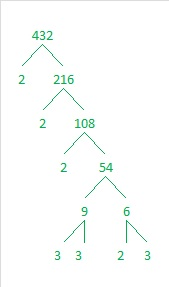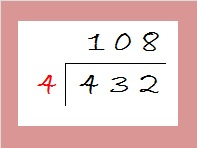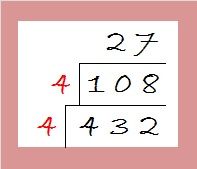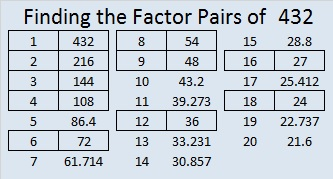# How to Simplify √432Many people use factor trees and prime factorizations to figure out how to simplify square roots. I don’t. I don’t see the point in breaking something completely apart just to put it back together again, especially when it ISN’T necessary to break it completely apart.

So instead of using a factor tree, I’ve modified the cake method, and I use it to simplify square roots.

For example, to find the square root of 500, I would never use its prime factorization: 500 = 2 x 2 x 5 x 5 x 5. Instead, I would first divide 500 by perfect square 100 to get 5. Then I would take the square root of both 100 and 5 to get 10√5.

Only 1% of numbers are divisible by 100, but for the ones that are, I always start by dividing by 100.

Now get this: Roughly 82.5% of all numbers that have simplifiable square roots can be evenly divided by perfect squares four and/or by nine. It is so easy to tell if a number can be evenly divided by either of those numbers. That is why I always start with one hundred, then four, then nine.

Let’s look at 432: Since the number formed from the last two digits, 32, is divisible by 4, I know that 432 is also divisible by four, so I will go ahead and do the division.Now I look at 108. The last two digits, 08, can also be evenly divided by 4, so I do that division as well:108 divided by 4 gives us 27 which is not divisible by 4. However since 2 + 7 = 9, I know that 27 is divisible by 9, so I do that division next:27 divided by 9 is 3. Since the only square number that will divide evenly into 3 is 1, I’m done with the division process. Now I take the square roots of all the numbers on the outside of the cake and multiply them together: √(4 x 4) x (√9) x (√3) = 4 x 3 x (√3) = 12√3.

I will give other examples of this method in future posts. Here’s today’s puzzle:Print the puzzles or type the factors on this excel file:10 Factors 2015-03-16

• 432 is a composite number.
• Prime factorization: 432 = 2 x 2 x 2 x 2 x 3 x 3 x 3, which can be written 432 = (2^4) x (3^3)
• The exponents in the prime factorization are 4 and 3. Adding one to each and multiplying we get (4 + 1)(3 + 1) = 5 x 4 = 20. Therefore 432 has exactly 20 factors.
• Factors of 432: 1, 2, 3, 4, 6, 8, 9, 12, 16, 18, 24, 27, 36, 48, 54, 72, 108, 144, 216, 432
• Factor pairs: 432 = 1 x 432, 2 x 216, 3 x 144, 4 x 108, 6 x 72, 8 x 54, 9 x 48, 12 x 36, 16 x 27, or 18 x 24
• Taking the factor pair with the largest square number factor, we get √432 = (√144)(√3) = 12√3 ≈ 20.7846•ivasallay